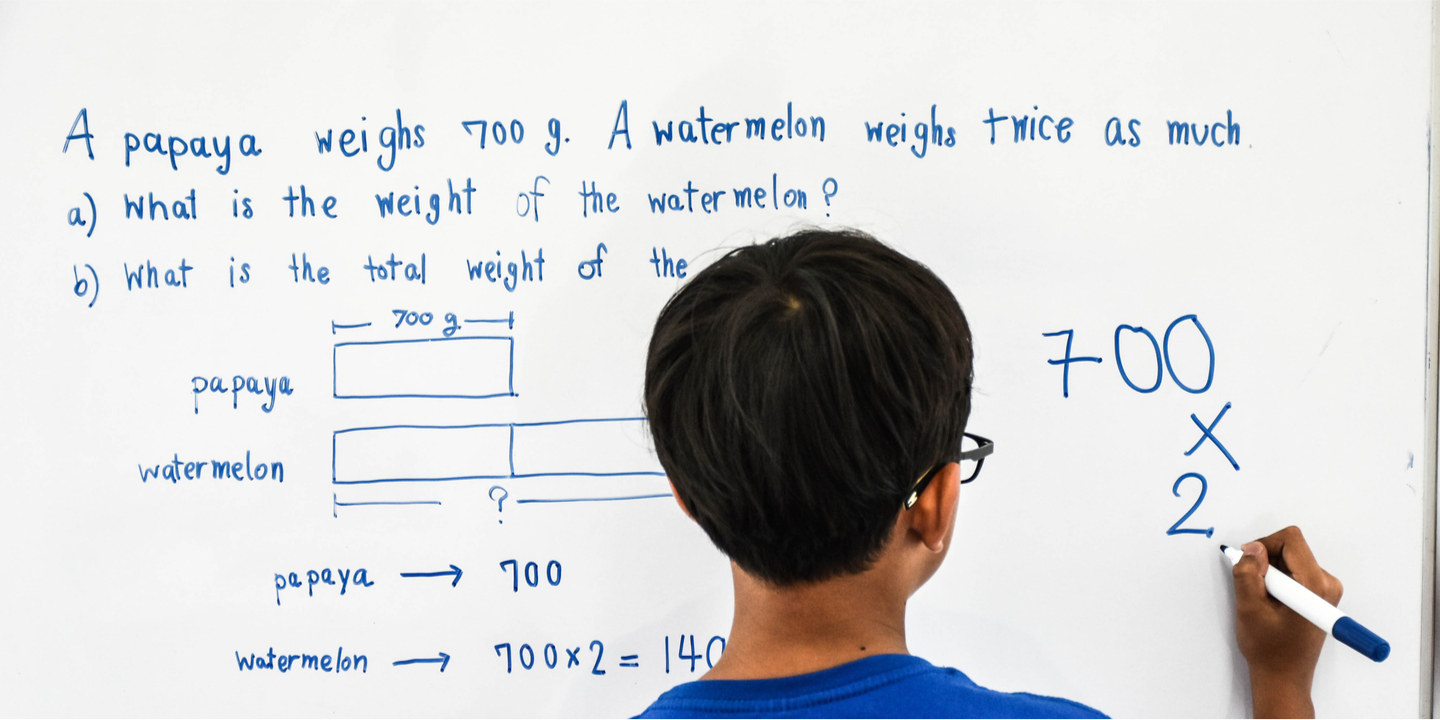Teaching numeracy

The nature of life and work in the 21st century means our school leavers need better numeracy and maths skills than ever before.

While numeracy and mathematics might seem like interchangeable terms, they are actually different. Numeracy is the ability to understand and use a range of mathematical and statistical knowledge and skills to solve real world problems.

A key to being numerate is to be able to start from real world problems or situations, excavate the maths out of the context and formulate it explicitly as a mathematical problem to solve. But evidence from large scale surveys suggests many school systems are not preparing students particularly well for mathematics in the real world.

A common approach in many maths classrooms is to teach some maths content, and then find an application where that maths can be used and applied. Unfortunately there are a couple of issues with that approach:

• the maths content is already specified and made explicit for them—so the student does not need to formulate the problem or decide on what maths to use
• the application can be in an unauthentic context or word problem, which can have a negative impact.

Four horses cost as much as three cows, four sheep as much as two horses, and three lambs as much as one sheep. How many cows could I exchange for 40 lambs?

Traditional school-based mathematical word problems such as this disregard and challenge students' sense making, and only serve to distance students from the real world, and the usefulness and value of mathematics.

If students have little experience grappling with messy real-world situations and problems, and if they can only apply mathematical procedures when problems are packaged in very familiar ways like in a classroom, then why would we expect them to be numerate and be able to see, use and apply maths in the world outside the classroom?

Numeracy therefore needs to be explicitly taught, through:

• problems set in authentic real world contexts
• explaining and demonstrating all the stages of mathematical modelling (formulating, employing mathematical knowledge and skills, interpreting/evaluating) which highlights how critical it is to recognise and excavate the maths out of a context
• a focus on using different mathematical processes (including communication, modelling, devising strategies, representation, and reasoning).

Teachers need to teach students how to read and interpret the context in which the maths is embedded, often in physical materials or in a printed or digital format. Moreover, in teaching numeracy you need to explicitly cover: a range of different cognitive processes and mathematical content areas; the use of appropriate tools and representations; and the skills required to reason, argue, reflect and evaluate, and then communicate the results.

A key part of teaching numeracy is to make the maths explicit and then, when the need arises, or gaps in mathematical knowledge appear, teach the maths that is required: 'just in time, not just in case'.

As a good starting point, instead of using traditional word problems of the kind shown above, teachers could utilise short realistic mathematics tasks more like those used in PISA. For extended learning, initiatives such as the International Mathematical Modeling Challenge provide students a deeper experience of how mathematics can explain our world and what working with mathematics looks like. ■

Find out more: